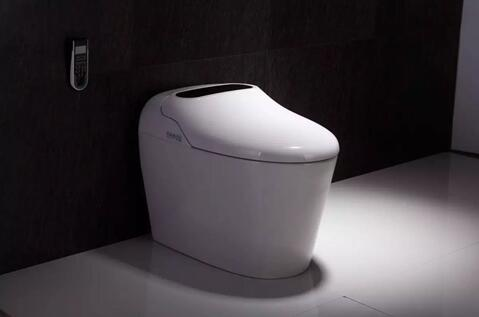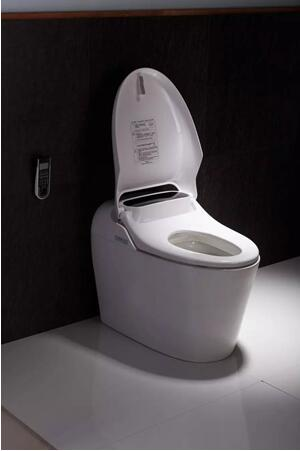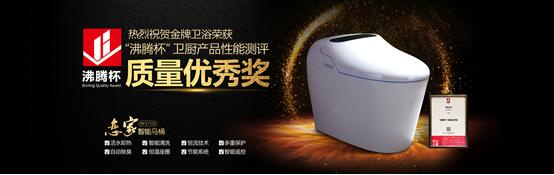|

# 智能马桶评测,拿奖的金牌RF3112Z又有什么升级

一款新产品的出现，有可能打开一个新时代的大门。

10年前，第一代苹果手机iPhone问世，谁会想到就是从它开始，定义了智能手机的模样。在智能马桶行业，正在重复同样的故事。

9月21日，金牌卫浴旗下的主力产品RF3112Z智能马桶，斩获首届“沸腾杯”智能马桶（坐便器）类品质大奖。此前，RF3112Z就引发了一定的关注，百度搜索该产品可查到近万条信息，知名媒体腾讯家居曾对RF3112Z做过一次测评，并连续发表《金牌卫浴再获殊荣，这款智能马桶您用上了吗？》《自从家里装了它，我再也没有躺在沙发上玩过王者荣耀了》等文章，另一家行业媒体中洁网更是刊文《你与“冠军”只差一款金牌智能马桶的距离》。

在这款智能马桶身上，我们看到了众多的改变与创新，甚至可以下一个结论，持续几年的智能马桶大战将进入中场赛。

值得注意的是，这些改变，并未局限于造型设计的创新与功能的升级，而是从用户体验、用户需求出发，解决传统智能马桶的痛点，带给居家生活的进一步改善。无水箱

与绝大多数智能马桶有明显区别的是，RF3112Z采用了无水箱设计，它的优点是相当明显的，没有水箱，自然不会存水，也就不会因为长期存水而产生污垢和细菌，使用清洗功能的时候，是活水冲洗，对业主来讲，自然更卫生。

另外，由于不带水箱，马桶占地面积更小，可以节省一定的卫浴空间，对于注重卫浴装修效果的业主来讲，RF3112Z可以让设计师的发挥空间更大，对提升卫浴间的颜值会有帮助。

一切为了方便

智能马桶之所以受到部分家庭的欢迎，一大关键原因就是它很方便。这些年来，占有一定市场份额的智能马桶品牌，基本上都有自己的方便卖点，RF3112Z更进了一步。

具体来讲，至少有三点方便做得很好：一个是超大LED显示屏，也就是我们常说的高清大屏，实时显示马桶的运行状态，方便用户浏览。二是带智能遥控器，触摸式按键，操控方便，还能防水。三是它的自动感应冲水相当灵敏，如厕后，座圈感应到人体压力，不用动手，冲水自动开启。

舒适为王

舒适往往是评判一款智能马桶好坏的关键标准。

RF3112Z在这方面的努力有目共睹，它的人体工程学设计，结合了大量用户的使用数据。它的即热式出水，消灭了清洗用水需要等待的痛点，也即清洗的时候，即开即热，打开就是热水，不需要等待，关键是不限时间，想洗多久都行，温度和水压还能根据自己的需要调节。

另外，针对此前别的品牌智能马桶在水温控制上出现水温不稳定，导致清洗的体验大打折扣的情况，金牌RF3112Z优化了PID控制算法，进一步提高了温度的精准，可以保持水温恒定。

再看它的清洗方式，可以个性化选择清洗的位置，还能前后摆动清洗。它的座圈舒适度也有提升，在25度-40度之间恒温，温度可以用智能遥控调节，选择你感觉最舒适的温度。不打扰

传统的智能马桶往往都具备一些静音功能，比如盖板的阻尼缓冲，RF3112Z则再进一步，强调不打扰、不影响，这种产品理念表现到具体功能上，既有盖板阻尼缓冲，也有对冲水声音大小的控制，同时还有对如厕异味的处理。

测评发现，对比大多数智能马桶，金牌RF3112Z的冲水声音有了明显的下降，在一个安静的空间里，几乎听不到明显的噪音。这样就确保了即使在夜里，也不会影响到家人的休息。另一方面，它本身带有自动除臭功能，化学触媒+活性炭吸附，能够自动吸走臭气，不破坏卫浴间的空气。

向健康看齐

一般的智能马桶，即使比较好的，大多不过是在功能的完善、静音、自动冲水、座圈加热及安全保护等性能上做文章，但金牌RF3112Z已经向健康看齐。

在这款产品上，金牌卫浴采用臂部清洗和女性清洗双管分离式，避免交叉污染。在使用前后，喷头会自动清洁，防止喷头不干净。待机时，挡板遮蔽喷管，避免外界污染。相当于做到了全流程的防污染保护，化解了大多数业主对喷头喷管不干净的担心。再者，它的暖风烘干设有三档，可调风口位置，满足不同用户的需求，用来预防痔疮、便秘等问题。即使在座圈这样的环节上，也考虑到了健康细节，比如增加了抗菌层。

“我见过很多智能马桶，部分还参加过测评，这次体验金牌RF3112Z之后，确实觉得智能马桶的技术又有进步了，相信技术越强大，功能越成熟，性能越稳定，以后会有更多的业主购买。”一位设计师表示。

`声明：本文由入驻焦点开放平台的作者撰写，除焦点官方账号外，观点仅代表作者本人，不代表焦点立场错误信息举报电话： 400-099-0099，邮箱：jubao@vip.sohu.com，或点此进行意见反馈，或点此进行举报投诉。`A B C D E F G H J K L M N P Q R S T W X Y Z
A - B - C - D - E
• A
• 鞍山
• 安庆
• 安阳
• 安顺
• 安康
• 澳门
• B
• 北京
• 保定
• 包头
• 巴彦淖尔
• 本溪
• 蚌埠
• 亳州
• 滨州
• 北海
• 百色
• 巴中
• 毕节
• 保山
• 宝鸡
• 白银
• 巴州
• C
• 承德
• 沧州
• 长治
• 赤峰
• 朝阳
• 长春
• 常州
• 滁州
• 池州
• 长沙
• 常德
• 郴州
• 潮州
• 崇左
• 重庆
• 成都
• 楚雄
• 昌都
• 慈溪
• 常熟
• D
• 大同
• 大连
• 丹东
• 大庆
• 东营
• 德州
• 东莞
• 德阳
• 达州
• 大理
• 德宏
• 定西
• 儋州
• 东平
• E
• 鄂尔多斯
• 鄂州
• 恩施
F - G - H - I - J
• F
• 抚顺
• 阜新
• 阜阳
• 福州
• 抚州
• 佛山
• 防城港
• G
• 赣州
• 广州
• 桂林
• 贵港
• 广元
• 广安
• 贵阳
• 固原
• H
• 邯郸
• 衡水
• 呼和浩特
• 呼伦贝尔
• 葫芦岛
• 哈尔滨
• 黑河
• 淮安
• 杭州
• 湖州
• 合肥
• 淮南
• 淮北
• 黄山
• 菏泽
• 鹤壁
• 黄石
• 黄冈
• 衡阳
• 怀化
• 惠州
• 河源
• 贺州
• 河池
• 海口
• 红河
• 汉中
• 海东
• I
• J
• 晋中
• 锦州
• 吉林
• 鸡西
• 佳木斯
• 嘉兴
• 金华
• 景德镇
• 九江
• 吉安
• 济南
• 济宁
• 焦作
• 荆门
• 荆州
• 江门
• 揭阳
• 金昌
• 酒泉
• 嘉峪关
K - L - M - N - P
• K
• 开封
• 昆明
• 昆山
• L
• 廊坊
• 临汾
• 辽阳
• 连云港
• 丽水
• 六安
• 龙岩
• 莱芜
• 临沂
• 聊城
• 洛阳
• 漯河
• 娄底
• 柳州
• 来宾
• 泸州
• 乐山
• 六盘水
• 丽江
• 临沧
• 拉萨
• 林芝
• 兰州
• 陇南
• M
• 牡丹江
• 马鞍山
• 茂名
• 梅州
• 绵阳
• 眉山
• N
• 南京
• 南通
• 宁波
• 南平
• 宁德
• 南昌
• 南阳
• 南宁
• 内江
• 南充
• P
• 盘锦
• 莆田
• 平顶山
• 濮阳
• 攀枝花
• 普洱
• 平凉
Q - R - S - T - W
• Q
• 秦皇岛
• 齐齐哈尔
• 衢州
• 泉州
• 青岛
• 清远
• 钦州
• 黔南
• 曲靖
• 庆阳
• R
• 日照
• 日喀则
• S
• 石家庄
• 沈阳
• 双鸭山
• 绥化
• 上海
• 苏州
• 宿迁
• 绍兴
• 宿州
• 三明
• 上饶
• 三门峡
• 商丘
• 十堰
• 随州
• 邵阳
• 韶关
• 深圳
• 汕头
• 汕尾
• 三亚
• 三沙
• 遂宁
• 山南
• 商洛
• 石嘴山
• T
• 天津
• 唐山
• 太原
• 通辽
• 铁岭
• 泰州
• 台州
• 铜陵
• 泰安
• 铜仁
• 铜川
• 天水
• 天门
• W
• 乌海
• 乌兰察布
• 无锡
• 温州
• 芜湖
• 潍坊
• 威海
• 武汉
• 梧州
• 渭南
• 武威
• 吴忠
• 乌鲁木齐
X - Y - Z
• X
• 邢台
• 徐州
• 宣城
• 厦门
• 新乡
• 许昌
• 信阳
• 襄阳
• 孝感
• 咸宁
• 湘潭
• 湘西
• 西双版纳
• 西安
• 咸阳
• 西宁
• 仙桃
• 西昌
• Y
• 运城
• 营口
• 盐城
• 扬州
• 鹰潭
• 宜春
• 烟台
• 宜昌
• 岳阳
• 益阳
• 永州
• 阳江
• 云浮
• 玉林
• 宜宾
• 雅安
• 玉溪
• 延安
• 榆林
• 银川
• Z
• 张家口
• 镇江
• 舟山
• 漳州
• 淄博
• 枣庄
• 郑州
• 周口
• 驻马店
• 株洲
• 张家界
• 珠海
• 湛江
• 肇庆
• 中山
• 自贡
• 资阳
• 遵义
• 昭通
• 张掖
• 中卫

1室1厅1厨1卫1阳台

1
2
3
4
5

0
1
2

1

1

0
1
2
3报名成功，资料已提交审核A B C D E F G H J K L M N P Q R S T W X Y Z
A - B - C - D - E
• A
• 鞍山
• 安庆
• 安阳
• 安顺
• 安康
• 澳门
• B
• 北京
• 保定
• 包头
• 巴彦淖尔
• 本溪
• 蚌埠
• 亳州
• 滨州
• 北海
• 百色
• 巴中
• 毕节
• 保山
• 宝鸡
• 白银
• 巴州
• C
• 承德
• 沧州
• 长治
• 赤峰
• 朝阳
• 长春
• 常州
• 滁州
• 池州
• 长沙
• 常德
• 郴州
• 潮州
• 崇左
• 重庆
• 成都
• 楚雄
• 昌都
• 慈溪
• 常熟
• D
• 大同
• 大连
• 丹东
• 大庆
• 东营
• 德州
• 东莞
• 德阳
• 达州
• 大理
• 德宏
• 定西
• 儋州
• 东平
• E
• 鄂尔多斯
• 鄂州
• 恩施
F - G - H - I - J
• F
• 抚顺
• 阜新
• 阜阳
• 福州
• 抚州
• 佛山
• 防城港
• G
• 赣州
• 广州
• 桂林
• 贵港
• 广元
• 广安
• 贵阳
• 固原
• H
• 邯郸
• 衡水
• 呼和浩特
• 呼伦贝尔
• 葫芦岛
• 哈尔滨
• 黑河
• 淮安
• 杭州
• 湖州
• 合肥
• 淮南
• 淮北
• 黄山
• 菏泽
• 鹤壁
• 黄石
• 黄冈
• 衡阳
• 怀化
• 惠州
• 河源
• 贺州
• 河池
• 海口
• 红河
• 汉中
• 海东
• I
• J
• 晋中
• 锦州
• 吉林
• 鸡西
• 佳木斯
• 嘉兴
• 金华
• 景德镇
• 九江
• 吉安
• 济南
• 济宁
• 焦作
• 荆门
• 荆州
• 江门
• 揭阳
• 金昌
• 酒泉
• 嘉峪关
K - L - M - N - P
• K
• 开封
• 昆明
• 昆山
• L
• 廊坊
• 临汾
• 辽阳
• 连云港
• 丽水
• 六安
• 龙岩
• 莱芜
• 临沂
• 聊城
• 洛阳
• 漯河
• 娄底
• 柳州
• 来宾
• 泸州
• 乐山
• 六盘水
• 丽江
• 临沧
• 拉萨
• 林芝
• 兰州
• 陇南
• M
• 牡丹江
• 马鞍山
• 茂名
• 梅州
• 绵阳
• 眉山
• N
• 南京
• 南通
• 宁波
• 南平
• 宁德
• 南昌
• 南阳
• 南宁
• 内江
• 南充
• P
• 盘锦
• 莆田
• 平顶山
• 濮阳
• 攀枝花
• 普洱
• 平凉
Q - R - S - T - W
• Q
• 秦皇岛
• 齐齐哈尔
• 衢州
• 泉州
• 青岛
• 清远
• 钦州
• 黔南
• 曲靖
• 庆阳
• R
• 日照
• 日喀则
• S
• 石家庄
• 沈阳
• 双鸭山
• 绥化
• 上海
• 苏州
• 宿迁
• 绍兴
• 宿州
• 三明
• 上饶
• 三门峡
• 商丘
• 十堰
• 随州
• 邵阳
• 韶关
• 深圳
• 汕头
• 汕尾
• 三亚
• 三沙
• 遂宁
• 山南
• 商洛
• 石嘴山
• T
• 天津
• 唐山
• 太原
• 通辽
• 铁岭
• 泰州
• 台州
• 铜陵
• 泰安
• 铜仁
• 铜川
• 天水
• 天门
• W
• 乌海
• 乌兰察布
• 无锡
• 温州
• 芜湖
• 潍坊
• 威海
• 武汉
• 梧州
• 渭南
• 武威
• 吴忠
• 乌鲁木齐
X - Y - Z
• X
• 邢台
• 徐州
• 宣城
• 厦门
• 新乡
• 许昌
• 信阳
• 襄阳
• 孝感
• 咸宁
• 湘潭
• 湘西
• 西双版纳
• 西安
• 咸阳
• 西宁
• 仙桃
• 西昌
• Y
• 运城
• 营口
• 盐城
• 扬州
• 鹰潭
• 宜春
• 烟台
• 宜昌
• 岳阳
• 益阳
• 永州
• 阳江
• 云浮
• 玉林
• 宜宾
• 雅安
• 玉溪
• 延安
• 榆林
• 银川
• Z
• 张家口
• 镇江
• 舟山
• 漳州
• 淄博
• 枣庄
• 郑州
• 周口
• 驻马店
• 株洲
• 张家界
• 珠海
• 湛江
• 肇庆
• 中山
• 自贡
• 资阳
• 遵义
• 昭通
• 张掖
• 中卫• 手机• 分享
• 设计
免费设计
• 计算器
装修计算器
• 入驻
合作入驻
• 联系
联系我们
• 置顶
返回顶部